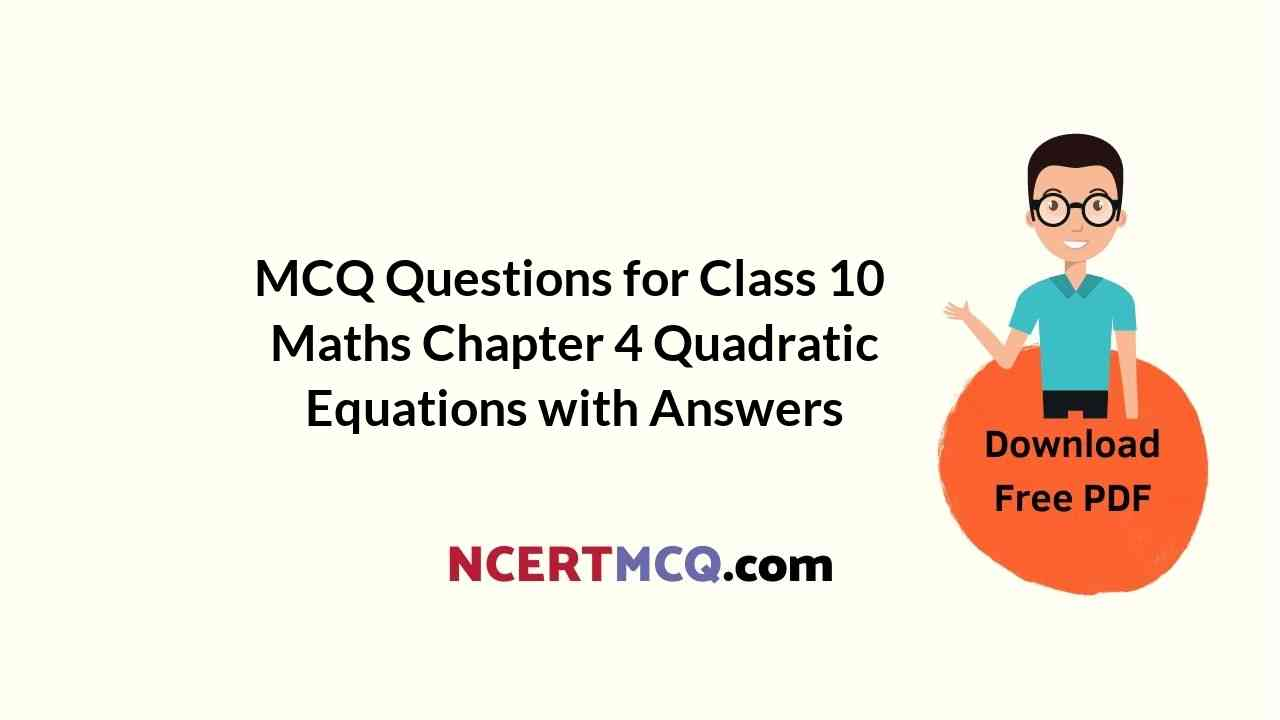Check the below NCERT MCQ Questions for Class 10 Maths Chapter 4 Quadratic Equations with Answers Pdf free download. MCQ Questions for Class 10 Maths with Answers were prepared based on the latest exam pattern. We have provided Quadratic Equations Class 10 Maths MCQs Questions with Answers to help students understand the concept very well. https://ncertmcq.com/mcq-questions-for-class-10-maths-with-answers/

Students can also refer to NCERT Solutions for Class 10 Maths Chapter 4 Quadratic Equations for better exam preparation and score more marks.

Quadratic Equation Class 10 MCQ Question 1.
Which of the following is a quadratic equation?
(a) x² + 2x+ 1 = (4 – x)² + 3
(b) -2x² = (5 – x)[2x – $$\frac{2}{5}$$]
(c) (k + 1)x² + $$\frac{3}{2}$$ x = 7, where k = -1
(d) x³ – x² = (x – 1)³

Answer: (d) x³ – x² = (x – 1)³

MCQ On Quadratic Equations Class 10 Question 2.
Which of the following is not a quadratic equation?
(a) 2(x – 1)² = 4x² – 2x + 1
(b) 2x – x² = x² + 5
(c) (√2x + √3)² + x² = 3x² – 5x
(d) (x² + 2x)² = x4 + 3 + 4x³

Answer: (c) (√2x + √3)² + x² = 3x² – 5x

Quadratic Equations Class 10 MCQ Question 3.
Which of the following equations has 2 as a root?
(a) x² – 4x + 5 = 0
(b) x² + 3x – 12 = 0
(c) 2x² – 7x + 6 = 0
(d) 3x² – 6x – 2 = 0

Answer: (c) 2x² – 7x + 6 = 0

Class 10 Maths Chapter 4 MCQ Question 4.
If $$\frac{1}{2}$$ is a root of the equation x² + kx – $$\frac{5}{4}$$ = 0 then the value of k is
(a) 2
(b) -2
(c) $$\frac{1}{4}$$
(d) $$\frac{1}{2}$$

MCQ Questions On Quadratic Equations For Class 10 Question 5.
Which of the following has the sum of its roots as 3?
(а) 2x² – 3x + 6 = 0
(b) -x² + 3x + 3 = 0
(c) √2x² – $$\frac{3}{√2}$$x + 1 = 0
(d) 3x² – 3x + 3 = 0

Answer: (b) -x² + 3x + 3 = 0

MCQ Questions For Class 10 Maths Quadratic Equations With Answers Pdf Question 6.
Values of k for which the quadratic equation 2x² – kx + k = 0 has equal roots is
(a) 0 only
(b) 4
(c) 8 only
(d) 0, 8

Quadratic Equations MCQs Class 10 Question 7.
Which constant must be added and subtracted to solve the quadratic equation 9x² + $$\frac{3}{4}$$ x – √2 = 0 by the method of completing the square?
(a) $$\frac{1}{8}$$
(b) $$\frac{1}{64}$$
(c) $$\frac{1}{4}$$
(d) $$\frac{9}{64}$$

Answer: (b) $$\frac{1}{64}$$

Class 10 Maths Chapter 4 MCQ With Answers Question 8.
The quadratic equation 2x² – √5x + 1 = 0 has
(a) two distinct real roots
(b) two equal real roots
(c) no real roots
(d) more than 2 real roots

MCQ Of Chapter 4 Maths Class 10 Question 9.
Which of the following equations has two distinct real roots?
(a) 2x² – 3√2x + $$\frac{9}{4}$$ = 0
(b) x² + x – 5 = 0
(c) x² + 3x + 2√2 = 0
(d) 5x² – 3x + 1 = 0

Answer: (b) x² + x – 5 = 0

MCQ Of Quadratic Equation Class 10 Question 10.
Which of the following equations has no real roots?
(a) x² – 4x + 3√2 = 0
(b) x² + 4x – 3√2 = 0
(c) x² – 4x – 3√2 = 0
(d) 3x² + 4√3 +4 = 0

Answer: (a) x² – 4x + 3√2 = 0

MCQ Quadratic Equations Class 10 Question 11.
(x² + 1)² – x² = 0 has
(a) four real roots
(b) two real roots
(c) no real roots
(d) one real roots